## Extra logarithmic factors

### Quick description

Many proofs in many areas of mathematics, but especially in parts of number theory and combinatorics, give rise to bounds that have logarithmic terms. This article is about why that should be, and gives some examples of proofs where they arise. So far it has just one example. It would be good to have many more, demonstrating many different reasons for the appearance of log factors.

### Example 1

An aligned rectangle is a rectangle in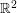with its sides parallel to theand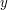axes. Suppose you have a collection ofaligned rectangles. What is the maximum value offor which it is always possible to find eitherdisjoint rectangles in the collection or a point inthat is contained inrectangles from the collection? For convenience we shall assume throughout the discussion that all rectangles are closed.

A simple example shows thatcannot be greater than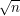: one simply takessets of aligned rectangles and makes sure that two aligned rectangles in the same set intersect, while two aligned rectangles in different sets are disjoint.

Now let us match this lower bound with an upper bound that is the same—apart from a logarithmic factor.

First, if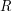is an aligned rectangle, let us define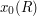and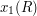to be the minimum and maximumcoordinates of points in, respectively. Similarly, let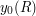and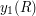be the minimum and maximumcoordinates. (Thus, ifis closed, then it equals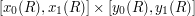. Now, given any collection ofaligned rectangles, let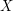be the set of all real numbers that are equal toorfor somein the collection, and let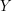be the same thing forcoordinates. Then the sizes ofandare both at most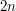.

Now whether or not two rectanglesand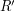intersect depends solely on the ordering of the four numbers,,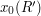and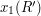and the ordering of the four numbers,,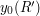and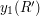. Therefore, we can replace the setsandby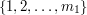and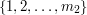for two integers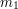and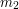that are both at most.

What all this amounts to is that we can assume, without loss of generality, that the coordinates of the vertices of all rectangles in the collection are integers betweenand.

Now it turns out to be a lot easier to prove the result if all the rectangles have about the same size and shape. Here is an argument that shows that gives a lower bound of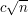for aligned squares. Let us form a graph whose vertices are the aligned squares, with an edge joining two vertices if those squares intersect. (If you are unfamiliar with graph theory terminology, then you can find it in Wikipedia's graph theory glossary.) If the maximum degree of any vertex in this graph is, then we can pick a sequence of vertices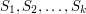, with no vertex joined to any previous one, provided that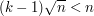, so certainly if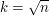. So in this case we have a collection ofdisjoint squares. But now suppose that there is a vertex of degree greater than. This will be a square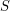that overlaps with overother squares. Now each of those other squares must contain a vertex of the original square (this is the step that fails when the rectangles have different shapes), so a least one vertex ofmust be contained in at least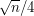rectangles, which completes the proof.

What can we do if we have rectangles? We can use the widely applicable trick of making everything roughly the same size. The widths of the rectangles lie betweenand, as do their heights. Let us partition the set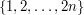into sets of the form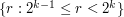. There are at most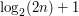of these sets, so we can find two of them,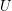and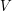say, and a set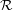of at least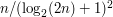aligned rectangles taken from the original collection, such that the width of every rectangle inlies inand the height lies in.

This implies that the ratios of the widths and heights of any two rectangles inare at most 2. We now repeat the argument above for squares, except that this time when we have a rectangleof degree at least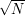(where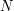is the size of), we cannot argue that all rectangles that intersectmust contain a vertex of. However, we can argue that any rectangle inthat intersectscontains either a vertex ofor the midpoint of an edge or the centre of. This makes nine possibilities, so we can obtain a lower bound of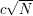. Sinceis of the form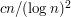, this gives a lower bound of the form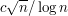for the original problem.

This demonstrates one way in which stray logarithmic factors can arise: through an application of the make-everything-roughly-the-same-size trick.

It is often possible to be more careful and remove a logarithmic factor of this kind by avoiding the use of that trick and thinking hard about how to relate objects of different sizes. However, closing the gap between the above estimates is still an open problem.

### Squares?

In the example proof, instead of squares, isn't it meant to be rectangles equal to each other? If squares are allowed to have different sizes, I don't think it is true that each neighbour of a square must overlap with one of its vertices.

A question: is there some reference for this problem and proof? I realize this problem asks for bounds on m in terms of n, where n=R(m,m) for intersection graph of rectangles. Was this studied for other special graphs?

The following comments were made inline in the article. You can click on 'view commented text' to see precisely where they were made.

### Only if squares are of the

Only if squares are of the same size, no? It would also work for rectangles with same sizes, right?

## Post new comment

(Note: commenting is not possible on this snapshot.)

Before posting from this form, please consider whether it would be more appropriate to make an inline comment using the Turn commenting on link near the bottom of the window. (Simply click the link, move the cursor over the article, and click on the piece of text on which you want to comment.)